Mean Deviation - Measures of Dispersion, Business Mathematics & Statistics

# Mean Deviation - Measures of Dispersion, Business Mathematics & Statistics - Business Mathematics and Statistics - B Com

Computation of Mean Deviation :

(a) For individual Observation (or Simple Variates)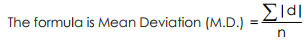Where | d | within two vertical lines denotes deviations from mean (or median), ignoring algebraic signs (i.e., + and –).

Coefficient of Mean Deviation :

Coefficient of Mean Deviation =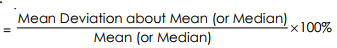Steps to find M. D.
(1) Find mean or median
(2) Take deviation ignoring ± signs
(3) Get total of deviations
(4) Divide the total by the number of items.

Example 45 : To find the mean deviation of the following data about mean and median : (`) 2, 6, 11, 14, 16, 19, 23.

Solution :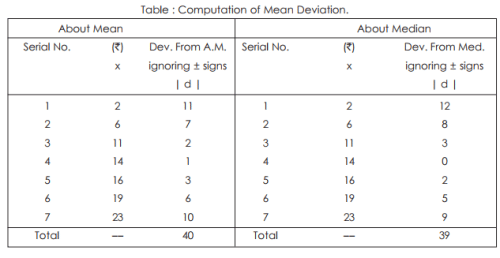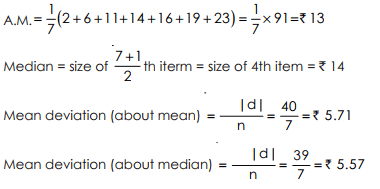Note : The sum of deviation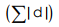about median is 39, less than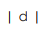about mean (= 40). Also M.D. about median.(i.e.5.57) is less than that about mean, (i.e., 5.71) Coefficient of Mean Deviation :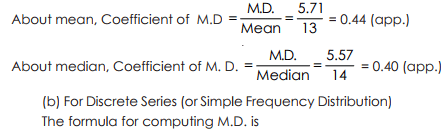(b) For Discrete Series (or Simple Frequency Distribution) The formula for computing M.D. is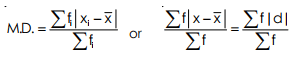Where= deviations from mean (or median) ignoring ± signs.

Steps of find M.D.
(i) Find weighed A.M. or median.
(ii) Find deviations ignoring ± signs. i.e.,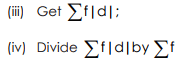Example 46 : To calculate mean deviation of the following series :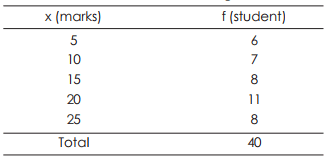Find also the coefficient of dispersion.
Solution :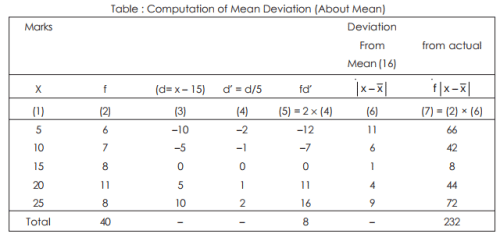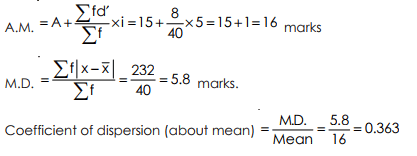Example 47 : The same example as given above.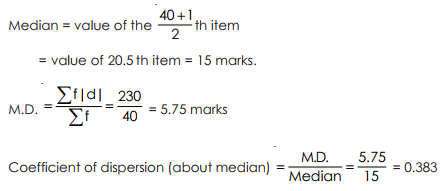(c) For Class Intervals (or Group Distribution) Steps to compute (M.D.)
(i) Find mid-value of the class intervals
(ii) Compute weighted A.M. or median
(iii) Findand f(iv) Divide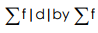Example 48 : Find M.D. about A.M. of the following frequency distribution.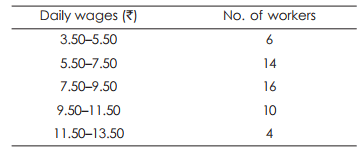Calculate also M.D. about median and hence find coefficient of mean dispersion.
Solution :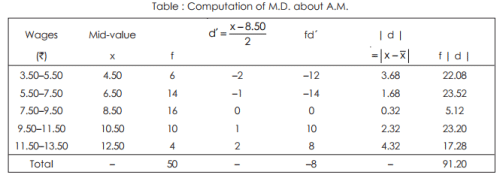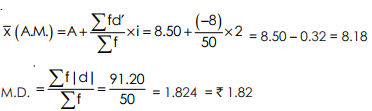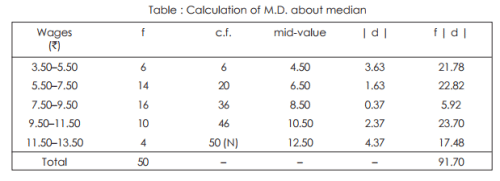Median = value of N/2th item = value of 50/2, i.e., 25 th item. So median class is (7.50 – 9.50)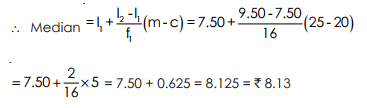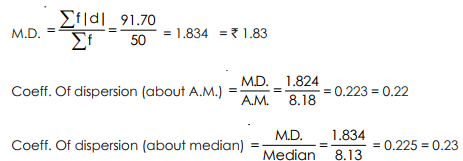(1) It is based on all the observations. Any change in any item would change the value of mean deviation.
(2) It is readily understood. It is the average of the deviation from a measure of central tendency.
(3) Mean Deviation is less affected by the extreme items than the standard deviation.
(4) It is simple to understand and easy to compute.

(1) Mean deviation ignores the algebraic signs of deviations and as such it is not capable of further algebraic treatment.
(2) It is not an accurate measure, particularly when it is calculated from mode.
(3) It is not popular as standard deviation.

Uses of Mean Deviation :

Because of simplicity in computation, it has drawn the attention of economists and businessmen. It is useful reports meant for public.

The document Mean Deviation - Measures of Dispersion, Business Mathematics & Statistics | Business Mathematics and Statistics - B Com is a part of the B Com Course Business Mathematics and Statistics.
All you need of B Com at this link: B Com

115 videos|142 docs

## FAQs on Mean Deviation - Measures of Dispersion, Business Mathematics & Statistics - Business Mathematics and Statistics - B Com

 1. What is the formula for calculating mean deviation?Ans. The formula for calculating mean deviation is as follows: Mean Deviation = Σ |X - μ| / N, where Σ represents the sum of all the absolute differences between each value (X) and the mean (μ), and N is the total number of values.
 2. How is mean deviation different from standard deviation?Ans. Mean deviation and standard deviation are both measures of dispersion, but they differ in terms of how they calculate the dispersion. Mean deviation measures the average distance of each value from the mean, while standard deviation measures the average deviation of each value from the mean, considering the squared differences. Standard deviation is more commonly used as it gives more weight to extreme values.
 3. What does mean deviation tell us about a data set?Ans. Mean deviation provides information about the spread or dispersion of a data set. A smaller mean deviation indicates that the values are closer to the mean, suggesting less variability. On the other hand, a larger mean deviation indicates greater variability and a wider spread of values from the mean.
 4. Can mean deviation be negative?Ans. No, mean deviation cannot be negative. The absolute values of the differences between each value and the mean are used in the calculation, ensuring that the result is always a positive value. The negative sign is disregarded when calculating the mean deviation.
 5. Is mean deviation affected by outliers in the data set?Ans. Yes, mean deviation is affected by outliers in the data set. Outliers, being extreme values, can significantly increase the mean deviation as they have larger differences from the mean. Therefore, mean deviation may not accurately represent the dispersion of the data when outliers are present. In such cases, other measures like median deviation or interquartile range may be more suitable.

115 videos|142 docsExplore Courses for B Com examSignup to see your scores go up within 7 days! Learn & Practice with 1000+ FREE Notes, Videos & Tests.
10M+ students study on EduRev
Track your progress, build streaks, highlight & save important lessons and more!
Related Searches

,

,

,

,

,

,

,

,

,

,

,

,

,

,

,

,

,

,

,

,

,

,

,

,

;